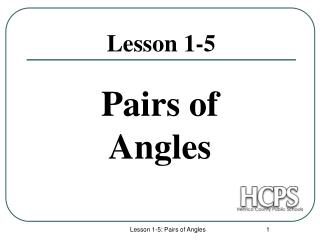DownloadDownload PresentationLesson 1-5

# Lesson 1-5

Download Presentation## Lesson 1-5

- - - - - - - - - - - - - - - - - - - - - - - - - - - E N D - - - - - - - - - - - - - - - - - - - - - - - - - - -
##### Presentation Transcript

1. Pairs of Angles Lesson 1-5 Lesson 1-5: Pairs of Angles

2. Adjacent Angles Definition: A pair of angles with a shared vertexand common sidebut do not have overlapping interiors. Examples: 1 and 2 are adjacent. 3 and 4 are not. 1 and ADC are not adjacent. 4 3 Adjacent Angles( a common side ) Non-Adjacent Angles Lesson 1-5: Pairs of Angles

3. Complementary Angles Definition: A pair of angles whose sum is 90˚ Examples: Adjacent Angles ( a common side ) Non-Adjacent Angles Lesson 1-5: Pairs of Angles

4. Supplementary Angles Definition: A pair of angles whose sum is 180˚ Examples: Adjacent supplementary angles are also called “Linear Pair.” Non-Adjacent Angles Lesson 1-5: Pairs of Angles

5. Vertical Angles Definition: A pair of angles whose sides form opposite rays. Examples: Vertical angles are non-adjacent angles formed by intersecting lines. Lesson 1-5: Pairs of Angles

6. Statements Reasons 1. 1. Definition: Linear Pair 2. 2. Property: Substitution 3. 3. Property: Subtraction 4. 4. Definition: Congruence Theorem: Vertical Angles are = ~ Given: The diagram Prove: Lesson 1-5: Pairs of Angles

7. What’s “Important” in Geometry? 4 things to always look for ! 90˚ 180˚ 360˚ Most of the rules (theorems) and vocabulary of Geometry are based on these 4 things. . . . andCongruence Lesson 1-5: Pairs of Angles

8. Example:If m4 = 67º, find the measures of all other angles. Step 1: Mark the figure with given info. Step 2: Write an equation. 67º Lesson 1-5: Pairs of Angles

9. Example: Ifm1 = 23 º and m2 = 32 º, find the measures of all other angles. Answers: Lesson 1-5: Pairs of Angles

10. Example: If m1 = 44º, m7 = 65º find the measures of all other angles. Answers: Lesson 1-5: Pairs of Angles

11. Algebra and Geometry Common Algebraic Equations used in Geometry: ( ) = ( ) ( ) + ( ) = ( ) ( ) + ( ) = 90˚ ( ) + ( ) = 180˚ If the problem you’re working on has a variable (x), then consider using one of these equations. Lesson 1-5: Pairs of Angles